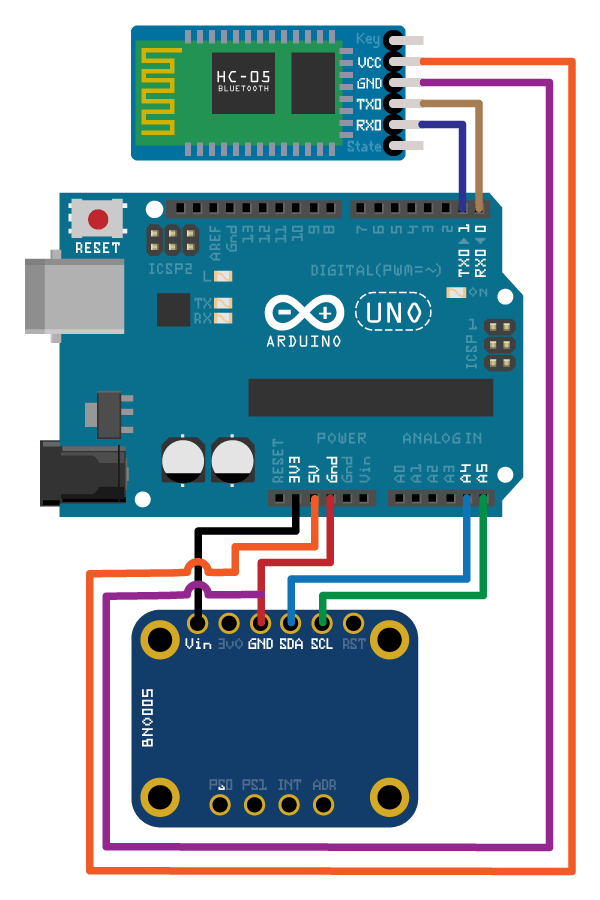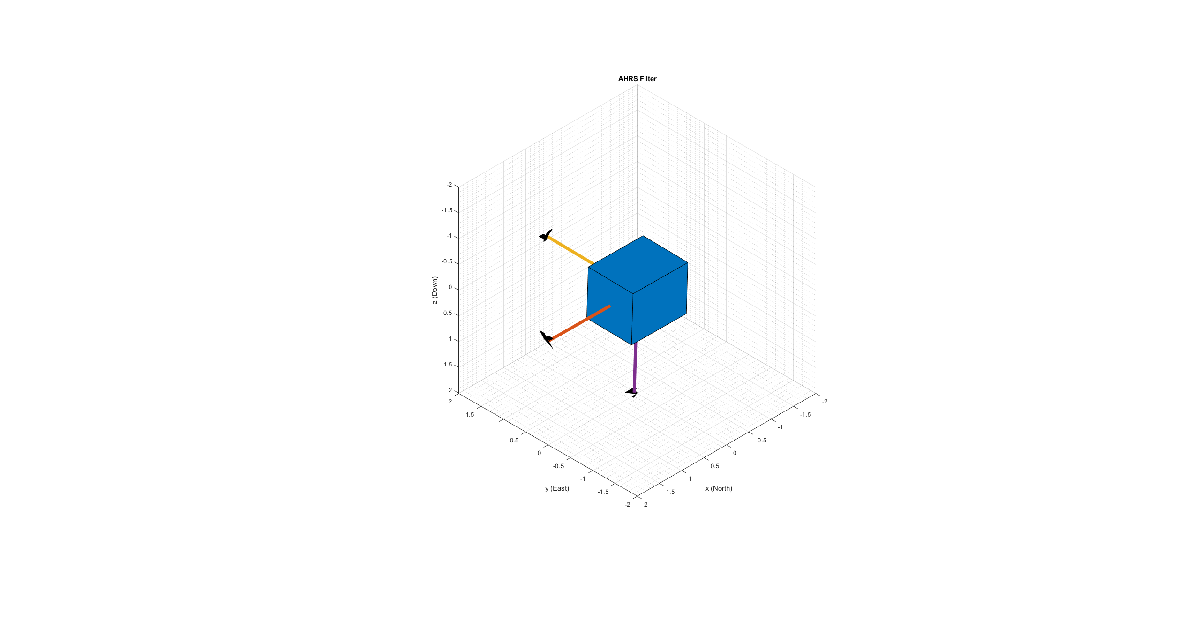# Wireless Data Streaming Using BNO055 and Bluetooth® and Estimating Orientation Using Sensor Fusion

This example shows how to get data from a Bosch BNO055 IMU sensor through HC-05 Bluetooth® module and to use the 9-axis AHRS fusion algorithm on the sensor data to compute orientation of the device. The example creates a figure which gets updated as you move the device.

BNO055 is a 9-axis sensor with accelerometer, gyroscope, and magnetometer. Accelerometer measures acceleration, gyroscope measures angular velocity, and magnetometer measures magnetic field in x-, y- and z- axes.

### Required MathWorks® Products

• MATLAB®

• MATLAB Support Package for Arduino® Hardware

• Instrument Control Toolbox™

• Sensor Fusion and Tracking Toolbox™ or Navigation Toolbox™

### Required Hardware

• Arduino® Uno

• Bosch BNO055 Sensor

• HC-05 Bluetooth® Module

### Hardware ConnectionConnect the SDA, SCL, GND, and the VCC pins of the BNO055 sensor to the corresponding pins on the Arduino® Uno board with the connections:

• SDA - A4

• SCL - A5

• VCC - +3.3V

• GND - GND

Connect the TX, RX, GND and VCC pins of the HC-05 module to the corresponding pins on the Arduino® Uno board. This example uses the connections:

• TX – Digital Pin 0 (RX)

• RX – Digital Pin 1 (TX)

• VCC – 5V

• GND – GND

Ensure that the connections to the sensors and Bluetooth® module are intact. It is recommended to use a BNO055 shield for Arduino Uno (Arduino 9 Axis Motion Shield). See Troubleshooting Sensors to debug the sensor related issues.

### Setup and Configure Arduino for Bluetooth® Communication

Configure the Arduino Uno board to communicate through Bluetooth® using the `arduinosetup`` `command from MATLAB command prompt. See Setup and Configure Arduino Hardware for steps on how to configure the Arduino board for communication through Bluetooth®. Make sure to check the box for I2C Libraries to be included during the Setup.

### Create Sensor Object

Create an `arduino` object.

`a = arduino('btspp://98D33230EB9F', 'Uno');`

Create the BNO055 sensor object.

``` fs = 100; % Sample Rate in Hz  imu = bno055(a,'SampleRate',fs,'OutputFormat','matrix'); ```

### Compensating For Hard Iron and Soft Iron Distortions

Fusion algorithms use magnetometer readings which need to be compensated for magnetic distortions such as hard iron distortion. Hard iron distortions are produced by materials which create a magnetic field, resulting in shifting the origin on the response surface. These distortions can be corrected by subtracting the correction values from the magnetometer readings for each axis. In order to find the correction values,

1. Rotate the sensor from 0 to 360 degree along each axis.

2. Use the magcal function to obtain the correction coefficients.

These correction values change with the surroundings.

To obtain correction coefficients for both hard iron and soft iron distortions:

```ts = tic; stopTimer = 50; magReadings=[]; while(toc(ts) < stopTimer) % Rotate the sensor along x axis from 0 to 360 degree. % Take 2-3 rotations to improve accuracy. % For other axes, rotate along that axes. [accel,gyro,mag] = read(imu); magReadings = [magReadings;mag]; end [A, b] = magcal(magReadings); % A = 3x3 matrix for soft iron correction % b = 3x1 vector for hard iron correction ```

### Aligning the axis of BNO055 sensor with NED Coordinates

Sensor Fusion algorithms used in this example use North-East-Down(NED) as a fixed, parent coordinate system. In the NED reference frame, the x-axis points north, the y-axis points east, and the z-axis points down. Depending on the algorithm, north may either be the magnetic north or true north. The algorithms in this example use the magnetic north. The algorithms used here expect all the sensors in the object to have their axes aligned with NED convention. The sensor values have to be inverted so that they are in accordance with the NED cordinates.

### Tuning Filter Parameters

The algorithms used in this example, when properly tuned, enable estimation of orientation and are robust against environmental noise sources. You must consider the situations in which the sensors are used and tune the filters accordingly. See Tuning Filter Parameters for more details related to tuning filter parameters.

The example uses ahrsfilter to demonstrate orientation estimation. See, Determine Orientation Using Inertial Sensors for more details related to inertial fusion algorithms.

### Accelerometer-Gyroscope-Magnetometer Fusion

An attitude and heading reference system (AHRS) consists of a 9-axis system that uses an accelerometer, gyroscope, and magnetometer to compute the orientation of the device. The `ahrsfilter` produces a smoothly changing estimate of orientation of the device, while correctly estimating the north direction. The `ahrsfilter` has the ability to remove gyroscope bias and can also detect and reject mild magnetic jamming.

The following code snippets use `ahrsfilter` system object to determine the orientation of the sensor and create a figure that gets updated as you move the sensor. The initial position of the sensor should be such that the device x-axis is pointing towards magnetic north, the device y-axis is pointing to east and the device z-axis is pointing downwards. You could use a cellphone or compass to determine magnetic north.

```% GyroscopeNoise, AccelerometerNoise and MagnetometerNoise are determined from the BNO055 datasheet using the following formula % NoisePower = OutputNoisePowerDensityrms^2 * Bandwidth GyroscopeNoiseBNO055 = 3.05e-06; % GyroscopeNoise (variance value) in units of (rad/s)^2 AccelerometerNoiseBNO055 = 67.53e-06; % AccelerometerNoise (variance value)in units of (m/s^2)^2 MagnetometerNoiseBNO055 = 1; %MagnetometerNoise (variance value) in units of uT^2 viewer = HelperOrientationViewer('Title',{'AHRS Filter'}); FUSE = ahrsfilter('SampleRate',imu.SampleRate,'GyroscopeNoise',GyroscopeNoiseBNO055,'AccelerometerNoise',AccelerometerNoiseBNO055,'MagnetometerNoise',MagnetometerNoiseBNO055); stopTimer=10; ```

After executing the below code snippet, slowly move the sensor and check if the motion in the figure matches the motion of the sensor. Increase the `stopTimer` value, if you need to track the orientation for longer time.

```magx_correction = b(1); magy_correction = b(2); magz_correction = b(3); ts = tic; while(toc(ts) < stopTimer) [accel,gyro,mag] = read(imu); % Align coordinates in accordance with NED convention accel = [-accel(:,1), accel(:,2), accel(:,3)]; gyro = [gyro(:,1), -gyro(:,2), -gyro(:,3)]; mag = [(mag(:,1)-magx_correction), -(mag(:,2)- magy_correction), -(mag(:,3)-magz_correction)] * A; rotators = FUSE(accel,gyro,mag); for j = numel(rotators) viewer(rotators(j)); end end```

If the sensor is stationary, at the initial position where the device x-axis points to the magnetic north, the device y-axis points to the east and the device z-axis points downwards, the x-axis in the figure will be parallel to and aligned with the positive x-axis, the y-axis in the figure will be parallel to and aligned with the positive y-axis and the z-axis in the figure will be parallel to and aligned with the positive z-axis.### Clean Up

When the connection is no longer needed, release and clear the objects

```release(imu); delete(imu); clear;```

### Things to try

You can try this example with other sensors such as InvenSense MPU-6050, MPU-9250, and STMicroelectronics LSM9DS1.